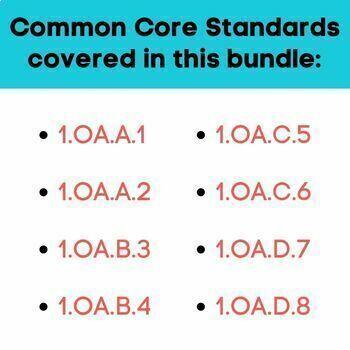# First Grade Common Core OA Worksheets GROWING BUNDLESubject
Resource Type
File Type

Zip

(26 MB|113 and more to come)
Product Rating
4.0
(1 Rating)
Standards
6 Products in this Bundle
6 products
• Bundle Description
• StandardsNEW

This bundle has everything you need to reinforce the fundamental math skills listed in the 1st grade Common Core Math Operations and Algebraic Thinking standards. The printables are kid-friendly to keep them engaging, not overwhelming. They can be used for classwork, math centers, homework, and even as assessments.

What is included?

1.OA.A.1
Use addition and subtraction within 20 to solve word problems involving situations of adding to, taking fro.m, putting together, taking apart, and comparing, with unknowns in all positions.

1.OA.A.2
Solve word problems that call for addition of three whole numbers whose sum is less than or equal to 20.

1.OA.B.3
Apply properties of operations as strategies to add and subtract.

1.OA.B.4
Understand subtraction as an unknown-addend problem.

1.OA.C.5
COMING SOON (FEBRUARY 5)

1.OA.C.6
COMING SOON (FEBRUARY12)

1.OA.D.7
Understand the meaning of the equal sign, and determine if equations involving addition and subtraction are true or false.

1.OA.D.8
Determine the unknown whole number in an addition or subtraction equation relating three whole numbers.

What is a growing bundle?

This means that the bundle is not completed yet. The price is lower as you are buying it knowing that I will create more quality materials for the bundle in a timely manner. After your initial purchase, you can download the updated files when I add the rest of the bundle.

What is the benefit of buying a growing bundle?

As more sets are added, the price will increase accordingly, which means you will be only paying a fraction of the final price if you buy it early. :)

Determine the unknown whole number in an addition or subtraction equation relating three whole numbers. For example, determine the unknown number that makes the equation true in each of the equations 8 + ? = 11, 5 = ▯ - 3, 6 + 6 = ▯.
Understand the meaning of the equal sign, and determine if equations involving addition and subtraction are true or false. For example, which of the following equations are true and which are false? 6 = 6, 7 = 8 - 1, 5 + 2 = 2 + 5, 4 + 1 = 5 + 2.
Add and subtract within 20, demonstrating fluency for addition and subtraction within 10. Use strategies such as counting on; making ten (e.g., 8 + 6 = 8 + 2 + 4 = 10 + 4 = 14); decomposing a number leading to a ten (e.g., 13 - 4 = 13 - 3 - 1 = 10 - 1 = 9); using the relationship between addition and subtraction (e.g., knowing that 8 + 4 = 12, one knows 12 - 8 = 4); and creating equivalent but easier or known sums (e.g., adding 6 + 7 by creating the known equivalent 6 + 6 + 1 = 12 + 1 = 13).
Relate counting to addition and subtraction (e.g., by counting on 2 to add 2).
Understand subtraction as an unknown-addend problem. For example, subtract 10 – 8 by finding the number that makes 10 when added to 8.
Total Pages
113 and more to come
Included
Teaching Duration
N/A
Report this Resource to TpT
Reported resources will be reviewed by our team. Report this resource to let us know if this resource violates TpT’s content guidelines.